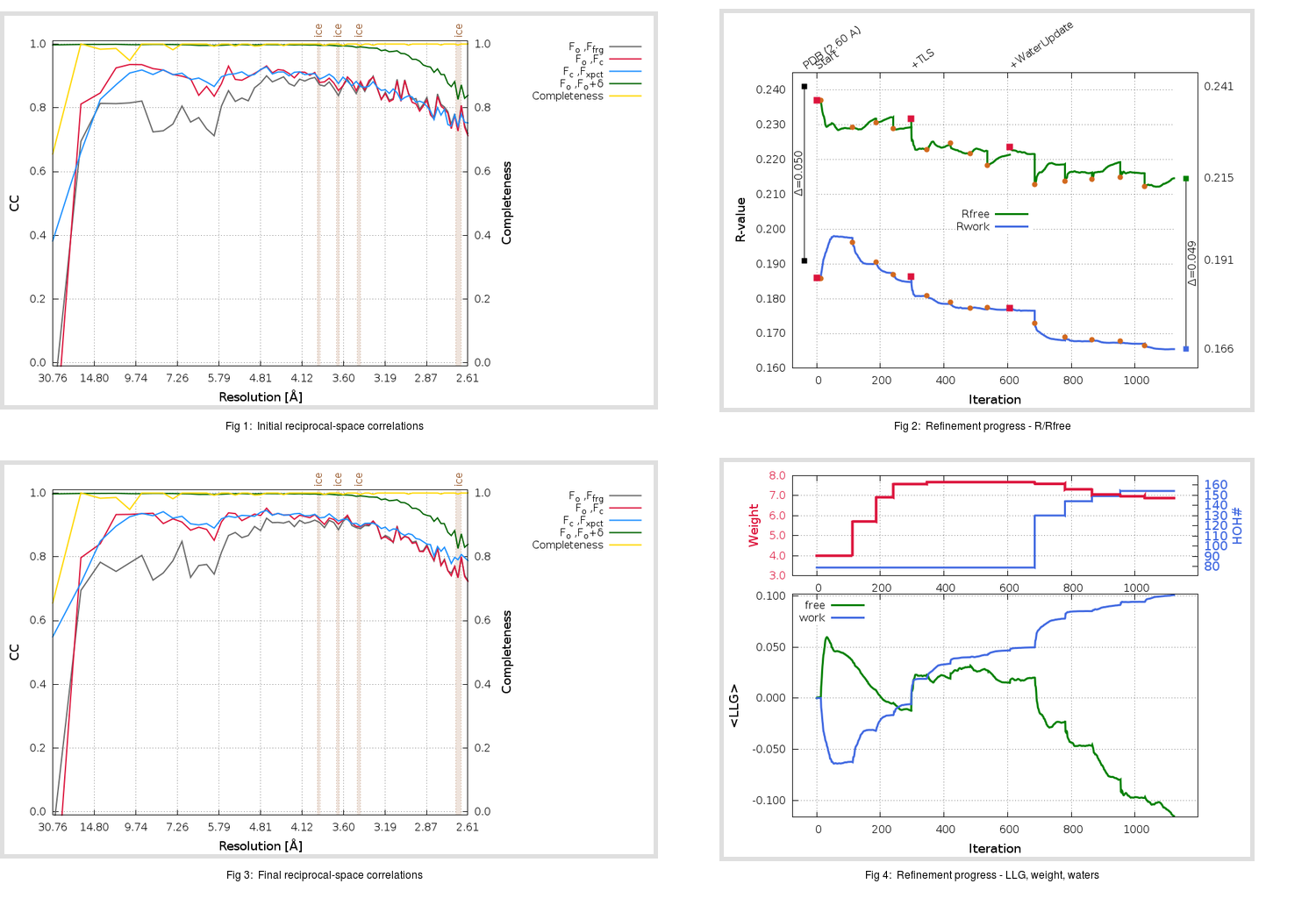Content:

## Deposited

` `
 Date deposited Date data collection Resolution R, Rfree 20110629 20081205 2.60 0.1880 0.2410

Molprobity (CCP4 7.0 version) summary:

```Ramachandran outliers =   0.33 %
favored =  96.08 %
Rotamer outliers      =   8.21 %
C-beta deviations     =     0
Clashscore            =   9.14
RMS(bonds)            =   0.0187
RMS(angles)           =   1.90
MolProbity score      =   2.45
Resolution            =   2.60
R-work                =   0.1880
R-free                =   0.2410
```

```Number of waters      =    79

<B> (all atoms) =   38.53 ( sd =   10.16 ) for       2509 non-hydrogen atoms
<B>   (protein) =   38.45 ( sd =   10.12 ) for       2406 non-hydrogen atoms
<B>     (water) =   36.43 ( sd =    9.16 ) for         79 non-hydrogen atoms
<B>    (others) =   55.68 ( sd =   14.84 ) for         24 non-hydrogen atoms

B min/max       (all non-hydrogen atoms) =   12.19 /   77.33
B min/max   (protein non-hydrogen atoms) =   13.32 /   77.31
B min/max     (water non-hydrogen atoms) =   12.19 /   60.27
B min/max     (other non-hydrogen atoms) =   41.78 /   77.33
```

## BUSTER (re-)refinement

` `

Molprobity (CCP4 7.0 version) summary:

```Ramachandran outliers =   0.33 %
favored =  96.73 %
Rotamer outliers      =   6.34 %
C-beta deviations     =     2
Clashscore            =   3.32
RMS(bonds)            =   0.0121
RMS(angles)           =   1.66
MolProbity score      =   1.94
Resolution            =   2.60
R-work                =   0.1655
R-free                =   0.2147
```

```Number of waters      =   154

<B> (all atoms) =   46.46 ( sd =   11.15 ) for       2584 non-hydrogen atoms
<B>   (protein) =   46.09 ( sd =   11.13 ) for       2406 non-hydrogen atoms
<B>     (water) =   49.68 ( sd =    9.68 ) for        154 non-hydrogen atoms
<B>    (others) =   65.85 ( sd =   15.32 ) for         24 non-hydrogen atoms

B min/max       (all non-hydrogen atoms) =   24.22 /   95.31
B min/max   (protein non-hydrogen atoms) =   24.22 /   95.31
B min/max     (water non-hydrogen atoms) =   29.34 /   89.85
B min/max     (other non-hydrogen atoms) =   61.55 /   79.99
```

Refinement progression:Results:

` `
 File Remark 3SNE_aB_refine.01_03_refine.pdb.gz exact refinement commands are in header 3SNE_aB_refine.01_03_refine.mtz.gz including original deposited data and several re-refinement map coefficients 3SNE_aB_refine.01_03_BUSTER_model.cif.gz including any non-standard compound restraints 3SNE_aB_refine.01_03_BUSTER_refln.cif.gz